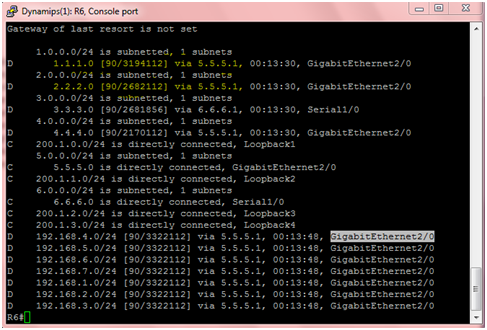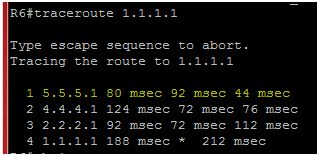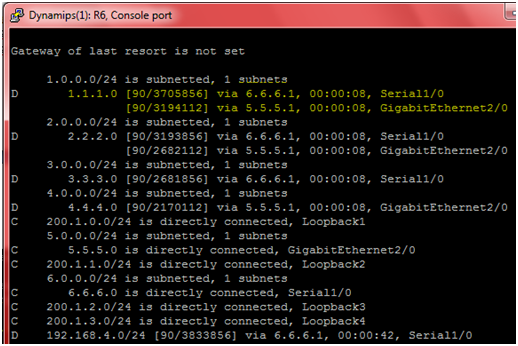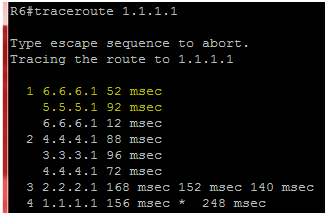EIGRP Unequal Cost Load Balancing configuration Gns3

EIGRP support both equal cost load balancing and unequal cost load balancing.
What is equal cost load balancing?
EIGRP by default support equal cost load balancing, across the same bandwidth links, EIGRP equally divide the traffic load to links.
What is unequal cost load balancing?
You can configure the unequal cost load balancing with EIGRP, in such case EIGRP divide the traffic according to bandwidth of links. This is unique feature of EIGRP, this is very useful, you can fully utilized your network resources. E.g. in our topology u can see from R6 there are two unequal links/ways to reach on R3, R2 and R1.Tracerroute also verifying that by default it is using only one link to reach 1.1.1.0 network.EIGRP Lab Configuration:
You can configure the R6 to use both serial and GigabitEthernet2 links for unequal cost load balancing by using only a single command.
Create and configure the above lab in GNS3 , for configuration you can visit EIGRP Configuration LAB on GNS3. After configuration use the following command to enable unequal cost load balancing.

Unequal cost load balancing command:
R6#config t
R6(config)#router eigrp 100
R6(config-router)#variance 3
Similarly you can also configure R4 and R5 for unequal cost load balancing.
Unequal cost load balancing Verifications:
R6#Show ip route
Now you will find the two path for each network with different matrix value.R6#traceroute 1.1.1.1 you will see that packet are following two paths to reaching R1.Similarity you can enable unequal cost load balancing on R4 and R5 and can verify with same commands.
Redistribute static route into EIGRP

UA-23728446-1